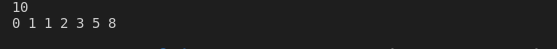Write a C++ program that takes a number from the user as an input and then print the Fibonacci series.

Program Sample

Sample Input

10

Sample Output

0 1 1 2 3 5 8

#include <iostream>
using namespace std;

int main()
{
// Febonacci Series
int number1 = 0, number2 = 1, nextterm = 0, number;
cin>>number;
// Using While Loop
// while(nextterm <= number)
// {
// cout<<nextterm<<" ";
// number1 = number2;
// number2 = nextterm;
// nextterm = number1 + number2;
// }

// By using for loop
for (nextterm =0 ;nextterm <= number; )
{
cout<<nextterm<<" ";
number1 = number2;
number2 = nextterm;
nextterm = number1 + number2;
}
return 0;
}c++ programming exercise with solution